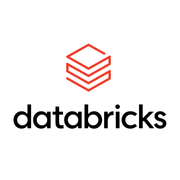Об этом курсе

Недавно просмотрено: 6 066
Гибкие сроки
Назначьте сроки сдачи в соответствии со своим графиком.
Сертификат, ссылками на который можно делиться с другими людьми
Получите сертификат по завершении
100% онлайн
Начните сейчас и учитесь по собственному графику.
Начальный уровень

S​ome experience with Data Science using the PyData Stack of NumPy, SciPy, Pandas, Scikit-learn.

Knowledge of Jupyter Notebooks will be beneficial.

Прибл. 13 часов на выполнение
Английский

Чему вы научитесь

• The basics of Probability, Bayesian statistics, modeling and inference.

• You will also get a hands-on introduction to using Python for computational statistics using Scikit-learn, SciPy and Numpy.

Приобретаемые навыки

• Bayesian Inference
• visualization
• Python Programming
• Scipy
• Statistics
Гибкие сроки
Назначьте сроки сдачи в соответствии со своим графиком.
Сертификат, ссылками на который можно делиться с другими людьми
Получите сертификат по завершении
100% онлайн
Начните сейчас и учитесь по собственному графику.
Начальный уровень

S​ome experience with Data Science using the PyData Stack of NumPy, SciPy, Pandas, Scikit-learn.

Knowledge of Jupyter Notebooks will be beneficial.

Прибл. 13 часов на выполнение
Английский

от партнераНеделя
1

Неделя 1

21 минута на завершение

Environment Setup

21 минута на завершение
4 видео ((всего 11 мин.)), 1 материал для самостоятельного изучения
Неделя
2

Неделя 2

6 ч. на завершение

Introduction to the Fundamentals of Probability

6 ч. на завершение
17 видео ((всего 119 мин.)), 7 материалов для самостоятельного изучения, 12 тестов
Неделя
3

Неделя 3

4 ч. на завершение

A Hands-On Introduction to Common Distributions

4 ч. на завершение
12 видео ((всего 49 мин.)), 2 материалов для самостоятельного изучения, 2 тестов
Неделя
4

Неделя 4

3 ч. на завершение

Sampling Algorithms

3 ч. на завершение
6 видео ((всего 32 мин.)), 2 материалов для самостоятельного изучения, 3 тестов

Специализация Introduction to Computational Statistics for Data Scientists: общие сведенияЧасто задаваемые вопросы

Остались вопросы? Посетите Центр поддержки учащихся.